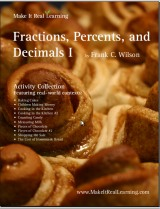May SALE! Get Math Mammoth and Make It Real Learning downloads & CDs at 25% off — right here at the MathMammoth.com website. Use coupon code WD2Q-P5CB-TXH4 at checkout. Offer valid through June 1, 2022.

^
You are here: HomeMake It Real Learning → Fractions, Decimals, Percents I

# Make It Real Learning Fractions, Decimals, Percents I workbook46 pages
(10 activities)

Free Sample (PDF):
Cooking in the Kitchen: Multiplying Fractions

# Introduction

The Make It Real Learning Fractions, Decimals, Percents I workbook focuses on real-world situations that may be effectively analyzed using proportional reasoning concepts such as fractions, percents, and unit conversion. This book suits best grades 4, 5, 6, 7, and 8.

From figuring out how much money is saved on a sale to determining how much of each ingredient to use when doubling a favorite cookie recipe, learners get to use basic math skills in meaningful ways. Each activity integrates real world information, companies, and issues (e.g. Aeropostale, Hershey's, cooking, baking, measuring milk, shopping), not just "realistic" data.

There are multiple ways to use the activities of the book in a teaching environment. The activities are an excellent tool for stimulating mathematical discussions in a small group setting. Due to the challenging nature of each activity, group members are motivated to brainstorm problem solving strategies together. The interesting real world contexts motivate them to want to solve the problems. The activities may also be used for individual projects and class-wide discussions.

As a ready-resource for teachers, the workbook also includes completely worked-out solutions for each activity. To make it easier for teachers to assess student work, the solutions are included on a duplicate copy of each activity.

The mathematical objectives of the activities fall within the standards of the National Council of Teachers of Mathematics.

## Fractions, Decimals, Percents I Activity List

Activity TitleMathematical Objectives
Baking Cakes:
Multiplying Fractions
Multiply a whole number by a fraction
Multiply two fractions
Convert improper fractions into mixed numbers
Children Making Money:
Working with Fractions
Create ratios
Simplify fractions
Compare two fractions and determine which is largest
Cooking in the Kitchen:
Multiplying Fractions
Multiply and divide fractions
Change units of measure through dimensional analysis
Cooking in the Kitchen #2:
Multiplying and Dividing Fractions
Multiply and divide fractions
Change units of measure through dimensional analysis
Counting Candy:
Using Pie Charts
Create a pie chart to represent a data set
Use a fraction to represent the shaded portion of a pie chart
Convert a fraction to a percent
Simplify a fraction
Measuring Milk:
Changing Units of Measure
Change units of measure through dimensional analysis
Multiply fractions
Write improper fractions as mixed numbers
Pieces of Chocolate:
Write a fraction that represents the shaded portion of a region
Shade the portion of a figure that is represented by a fraction
Add and subtract fractions with different denominators
Write improper fractions as mixed numbers
Pieces of Chocolate #2:
Write a fraction that represents the shaded portion of a region
Shade the portion of a figure that is represented by a fraction
Add and subtract fractions with different denominator
Shopping the Sale:
Using Percents
Convert percents into decimals and vice versa
Increase numbers by a constant percentage
Solve linear equations
Use ratios to find percents
with Fractions, Decimals, and Units
Multiply two fractions
Convert fractions to decimals
Change units of measure using dimensional analysis

Bundle specials!

Activity Library, Volume I. Price: \$39.99
Includes 11 Make It Real Learning activity workbooks for the price of 8! You get three workbooks for free!

Activity Library, Volume II. Price: \$39.99
Includes 11 Make It Real Learning activity workbooks for the price of 8! You get three workbooks for free!

States by the Numbers Bundle. Price: \$19.99
Inludes all 50 States by the Numbers workbooks at a whopping 87% discount!
(Normal price would be 50 × \$2.99 = \$149.50)

By purchasing any of the Make It Real Learning workbooks, permission IS granted for the teacher (or parent) to reproduce this material to be used with his/her students in a teaching situation; not for commercial resale. However, you are not permitted to share the material with another teacher.

In other words, you are permitted to make copies for the students/children you are teaching, but not for other teachers' usage.

WAIT!

Receive my monthly collection of math tips & resources directly in your inbox — and get a FREE Math Mammoth book!You can unsubscribe at any time.

### Math Mammoth Tour

Confused about the different options? Take a virtual email tour around Math Mammoth! You'll receive:

An initial email to download your GIFT of over 400 free worksheets and sample pages from my books. Six other "TOURSTOP" emails that explain the important things and commonly asked questions concerning Math Mammoth curriculum. (Find out the differences between all these different-colored series!)

This way, you'll have time to digest the information over one or two weeks, plus an opportunity to ask me personally about the curriculum.
A monthly collection of math teaching tips & Math Mammoth updates (unsubscribe any time)### "Mini" Math Teaching Course

This is a little "virtual" 2-week course, where you will receive emails on important topics on teaching math, including:

- How to help a student who is behind
- Troubles with word problems
- Teaching multiplication tables
- Why fractions are so difficult
- The value of mistakes
- Should you use timed tests
- And more!

A GIFT of over 400 free worksheets and sample pages from my books right in the very beginning.A monthly collection of math teaching tips & Math Mammoth updates (unsubscribe any time)
Enter your email to receive math teaching tips, resources, Math Mammoth news & sales, humor, and more! I tend to send out these tips about once monthly, near the beginning of the month, but occasionally you may hear from me twice per month (and sometimes less often).• A GIFT of over 400 free worksheets and sample pages from my books.